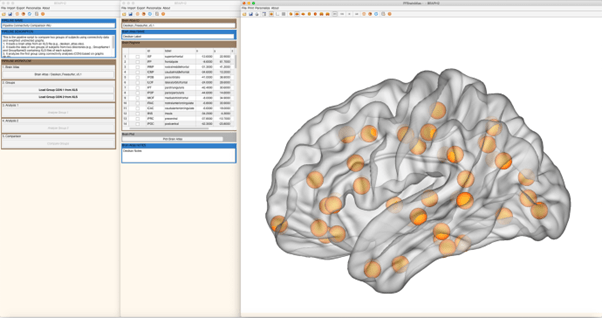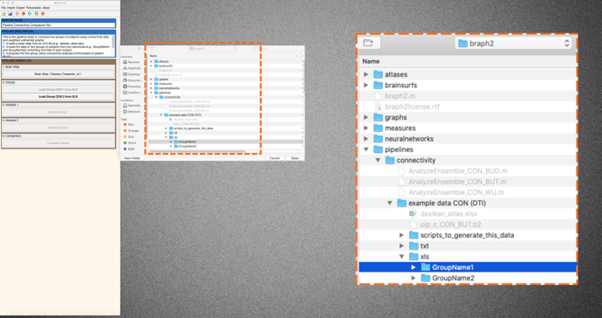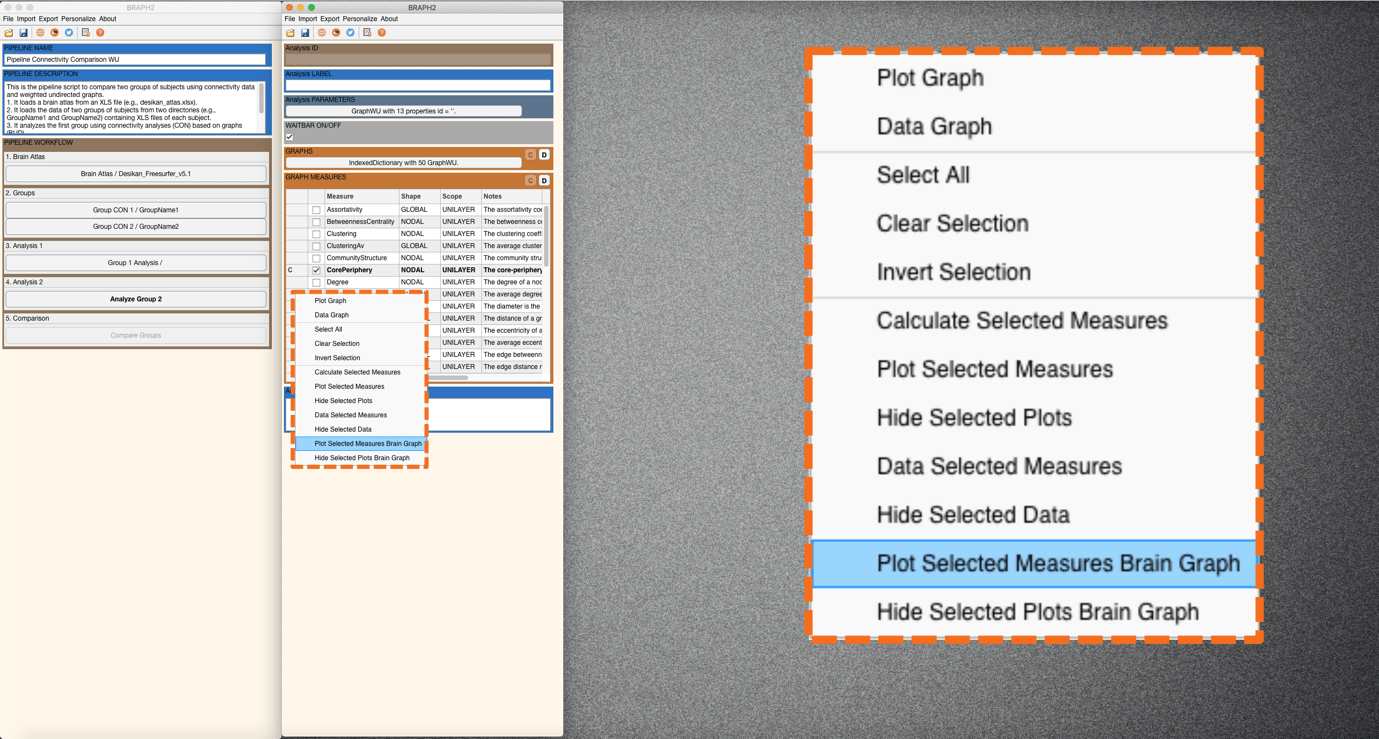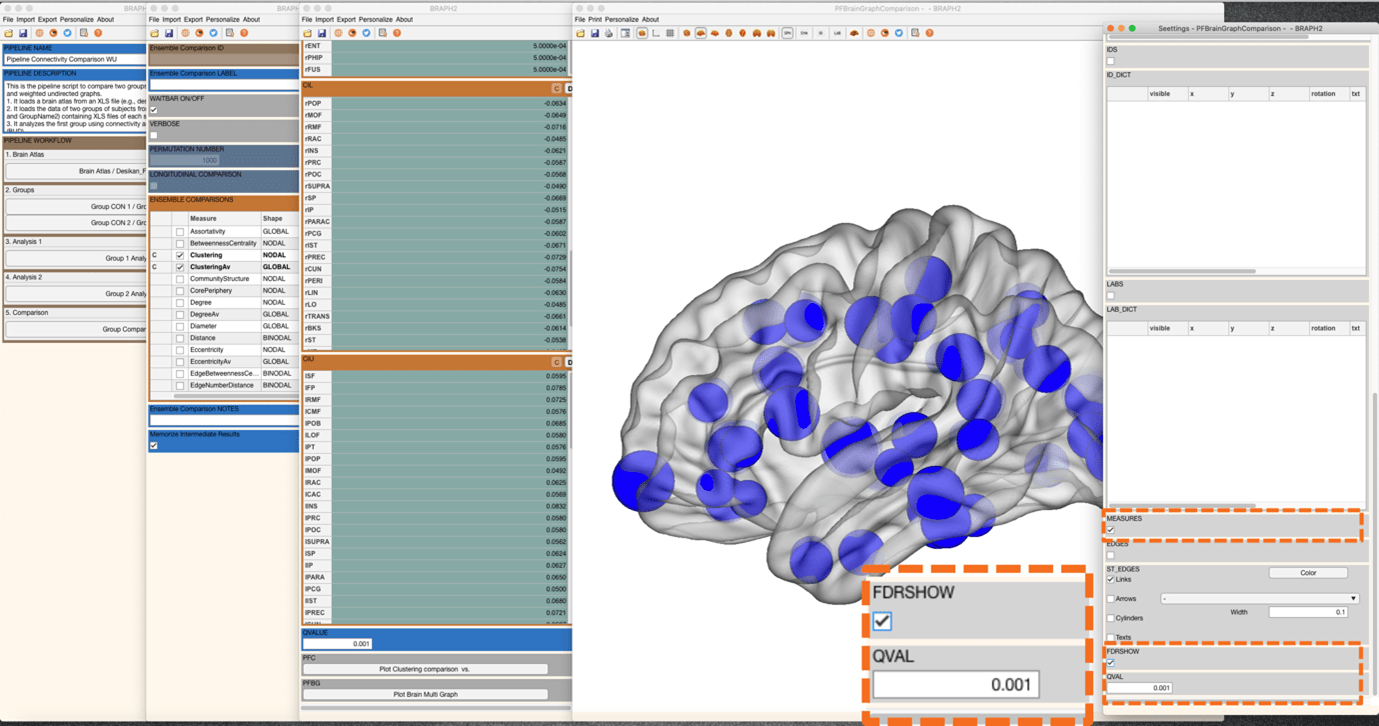# Pipeline Connectivity Comparison WU

## CON Comparison WU

Description:

This is the Graphical User Interface (GUI) tutorial for the pipeline that compares two groups of subjects using connectivity data (such as DWI) and weighted undirected graphs.

In this tutorial, we will use the example data, but you can use your own data as well (for instructions on how to prepare your own connectivity data to be analyzed in BRAPH2, see tutorial Connectivity Data format).

• Start BRAPH 2 and select the Pipeline from the main GUI

Start MATLAB, navigate to the BRAPH2 folder and run “braph2” with the following command:

>> braph2

Select the Pipeline Connectivity Comparison WU in the right menu. You can use the search field, typing connectivity for example (Figure 1).Figure 1. Landing GUI

Once you click on the pipeline, the window in Figure 2 will open.Figure 2. Landing pipeline GUI.The first step is to load a brain atlas from an XLS file. Press the button “Load a Brain Atlas XLS” (the only one active in the pipeline GUI, Figure 2) and then select the desikan_atlas.xlsx in the directory ./braph2/pipelines/connectivity/example data CON (DTI) (Figure 3).

Finally, to visualize the atlas press “Plot the brain atlas” and play with the surface settings (Figure 4). Check the Brain Atlas section for more information on how to control the appearance in this interface.Figure 4. Plot the brain atlas.Figure 5. From the pipeline GUI, first load data for group 1 (GroupName1) and then for group 2.

To load the data for the two groups you would like to compare press “Load Group CON 1 from XLS” and select the folder for subjects of Group1 which is at the directory ./braph2/pipelines/connectivity/example data CON (DTI)/xls/GroupName1 (Figure 5). After, load the data for group 2 which can be found at directory ./braph2/pipelines/connectivity/example data CON (DTI)/xls/GroupName2.

• Performing the connectivity analysis of group 1

Analysis of the first group using connectivity analyses (CON) based on weighted undirected graphs (WU).

First press “Analyze Group 1” to open the interface for its analysis (Figure 6). Then under GRAPHS section, by pressing “C” you can check the graphs for each subject. Maybe more interesting would be to plot the average graph for the whole group, for that go to section GRAPH MEASURES and press “C”, right-click on the left of a measure to deploy the menu seen in Figure 7 and select “Plot Graph”. We will see the weighted graph which is represented as an adjacency matrix. We could also play to plot this matrix when using different densities or thresholds, although in this tutorial we will proceed with the weighted analysis.Figure 6. After pressing Analyze group 1, the interface for Analysis of group 1 opens.Figure 7. Plot graph. By default, we see the weighted adjacency matrix.

Now it is the moment to calculate some connectivity measures for group 1. Select one measure, for example, Core Periphery (nodal), and calculate by right clicking on the left-side of the measures list and press “Calculate Selected Measures”. When the measure is calculated, a C appears on its left, and now, again by right-clicking on the left side of the measures list and pressing “Plot Selected Measures Brain Graph” (see Figure 8), we will be able to visualize the results of Core Periphery for group 1.Figure 8. When the measure is calculated, the C appears and then you can plot it on a brain surface.

A new figure with the brain surface will open with default settings for brain region visualization. If we go down in the Settings figure, we will find Measures panel. By checking the box, we can adapt the size and the color of the brain regions spheres to be proportional to the measure’s value (Figure 9), where blueish colors represent smaller values, and red larger. If we also check the box at EDGES panel, then the edges of the graph will be also on the plot (Figure 9).Figure 9. Checking the box at Measures and the box at Edges.

We can also first press “Data Selected Measures” from the same menu mentioned earlier (GRAPH MEASURES), and then a figure will open showing the array of values for the measure (Figure 10), and from there we can press “Plot Brain Graph” to visualize the results of Core Periphery for group 1 on a brain surface, so we will land to the same brain surface plot as before.Figure 10. Show the data of the selected measure.

In case we want to calculate a measure with different rules or parameters, it is done by going first to “Data Selected Measuresbefore calculating the measure. For more details, check the section Measures’ calculation with different parameters.

• Performing the connectivity analysis of group 2

Analysis of the second group using the same parameters selected for the first group (connectivity analyses (CON) based on weighted undirected graphs (WU)).

Follow the previous steps in the analysis of group 1 and explore some other measures for group 2.

• Comparing the two groups

To proceed with the group comparison press “Compare Groups” and the interface to select the comparison parameters will open (Figure 11). After setting the desired permutation number (recommended 1000 – 10000 permutations), press “C” under the ENSEMBLE COMPARISONS section to open the list of measures to use to compare the groups.Figure 11. Open the Group Comparison interface.

It is possible to select multiple measures to be compared, and then right click on the left of the measure table and select “Calculate Selected Comparisons” (Figure 12). Depending on how many permutations you use it will take less or more time. Moreover, in connectivity and functional analyses, it takes more time than in structural, since the measure is calculated per each subject’s network from the groups, and then it is averaged to perform the comparison.Figure 12. Calculation of the weighted comparison.

Once the calculations are done, you should see a C on the left of the measure to be compared (Figure 13). Then press “Data Selected Comparison” to show the difference values between groups and the associated p-values resulting from the permutation testing (Figure 13). Significant differences after FDR correction at the default value of q = 0.05 (q level can be changed at the panel QVALUE) are highlighted in the table by green. In this case, using the example data with weights, everything is significant.Figure 13. Show the results of the comparisons of multiple measures.

We can also plot the results from the comparison by pressing “Plot Clustering Comparison” for the global measures and “Plot Brain Multi Graph” for the nodal measures, from the GUI containing the data tables.

Finally, if we go down in the Settings figure, we will find FDR SHOW panel. By checking the box, just the regions that are significantly different between groups after applying FDR correction at the desired level (in Figure 14, the q value is set at 0.001) will be shown, the rest will be hidden. In this case, all differences are in the same direction, clustering is larger in group 1 than in group 2 (blue color indicates group 1 > group 2).Figure 14. Regions that are significant after applying FDR at a q_value = 0.001.